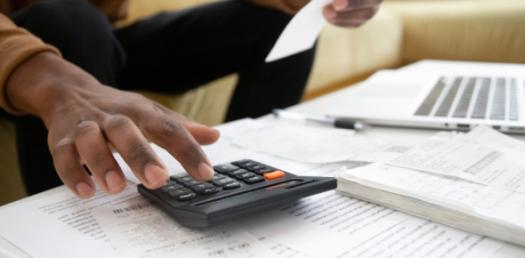# Quiz: Business Mathematics & Statistics #5

25 Questions | Attempts: 213
ShareSettings• 1.
If the price of an English novel decreases from Rs 255.50 to Rs 230.25. What is the percentage decrease?
• A.

8.9 %

• B.

9.9 %

• C.

10.9%

• D.

11.9 %

• 2.
Reduction from original selling Price is called …
• A.

Loss

• B.

List price

• C.

Profit

• D.

Markdown

• 3.
Write a formula to find the simple interest (I) if \$5000 is invested at 4% for 2 years.
• A.

I=PRT

• B.

I=P+R+T

• C.

I=PT

• D.

I=P(R+T)

• 4.
After marketing analysis, the Contribution Margin in rupees provided that  variable cost = Rs.400 & expected sale price is Rs.800 is:
• A.

2

• B.

1/2

• C.

-400

• D.

400

• 5.
The world is getting warmer — and U.S. scientists now know precisely how much warmer. They calculated the average percentage rise of 0.015% at the end of the century. If the current average temperature of the planet is X, what would be the average temperature of the earth at the end of 4008 AD?
• A.

X(1+0.015)^2

• B.

X(1+0.015%)^2

• C.

2(1+)^0.015

• D.

X(1+X)0.015

• 6.
If the basic salary of an employee is 8, 000 Rs. allowances are 6000 Rs. and total cost of leaves per year is equal to 28280 Rs. then the cost of leaves as percent of gross salary is
• A.

29.46%

• B.

16.83%

• C.

22.23%

• D.

28.28%

• 7.
If the interest rate 12%  and the number of payments are 15, then the accumulated factor is -----------------.
• A.

32.28

• B.

34.28

• C.

35.28

• D.

37.28

• 8.
Stock represents a claim on the company's:
• A.

Assets

• B.

Earning

• C.

Asset and earning

• D.

Share

• 9.
The negative of the negative of an odd number is:
• A.

Negative

• B.

positive

• C.

The odd number

• D.

Does not exist

• 10.
3x2 + 5x – 7 is ---------------   expression.
• A.

Monomial

• B.

Binomial

• C.

Trinomial

• D.

Linear

• 11.
If a and b are any two integers such that a:
• A.

Positive

• B.

Negative

• C.

Canot be told

• D.

Zero

• 12.
Which is the no when it multiply by 57 answer will be 1?
• A.

1/57

• B.

-57

• C.

1

• D.

none

• 13.
The short cut method of showing a number is multiplied by itself is called:
• A.

Superscript

• B.

Subscript

• C.

Exponent

• D.

None of these

• 14.
Net price =
• A.

• B.

• C.

• D.

• 15.
If a matrix has four column and 5 rows then its dimensions are:
• A.

20

• B.

4x5

• C.

5x4

• D.

5x5

• 16.
Interest calculated upon the principal amount added to the interest on it is called…
• A.

Simple interest

• B.

Compound interest

• C.

Annual interest per year

• D.

Semi annual interest

• 17.
Anwar buys a pencil for Rs 3. He sells it for Rs 5. What is his Percent Markup on Selling Price?
• A.

20%

• B.

30%

• C.

40%

• D.

50%

• 18.
The value of x for the equation -(-x - 5) = x – 2  is ------------------
• A.

No solutions

• B.

-5

• C.

3

• D.

1 / 2

• 19.
If x: y = y: 1, then:
• A.

X = y

• B.

X = y^2 or X = y2

• C.

X = y / x

• D.

X = -y

• 20.
Reduction from original selling Price is called ………
• A.

Loss

• B.

List price

• C.

Profit

• D.

Markdown

• 21.
After marketing analysis, the Contribution Margin in rupees provided that  variable cost = Rs.400 & expected sale price is Rs.800 is:
• A.

2

• B.

1/2

• C.

-400

• D.

400

• 22.
If the basic salary of an employee is 8, 000 Rs. allowances are 6000 Rs. and total cost of leaves per year is equal to 28280 Rs. then the cost of leaves as percent of gross salary is:
• A.

29.46%

• B.

16.83%

• C.

22.23%

• D.

28.28%

• 23.
Stock represents a claim on the company's:
• A.

Assets

• B.

Earning

• C.

Asset and earning

• D.

Share

• 24.
3x2 + 5x – 7 is ---------------   expression.
• A.

Monomial

• B.

Binomial

• C.

Trinomial

• D.

Linear

• 25.
Which is the no when it multiply by 57 answer will be 1?
• A.

1/57

• B.

-57

• C.

1

• D.

none

## Related TopicsBack to top
×

Wait!
Here's an interesting quiz for you.